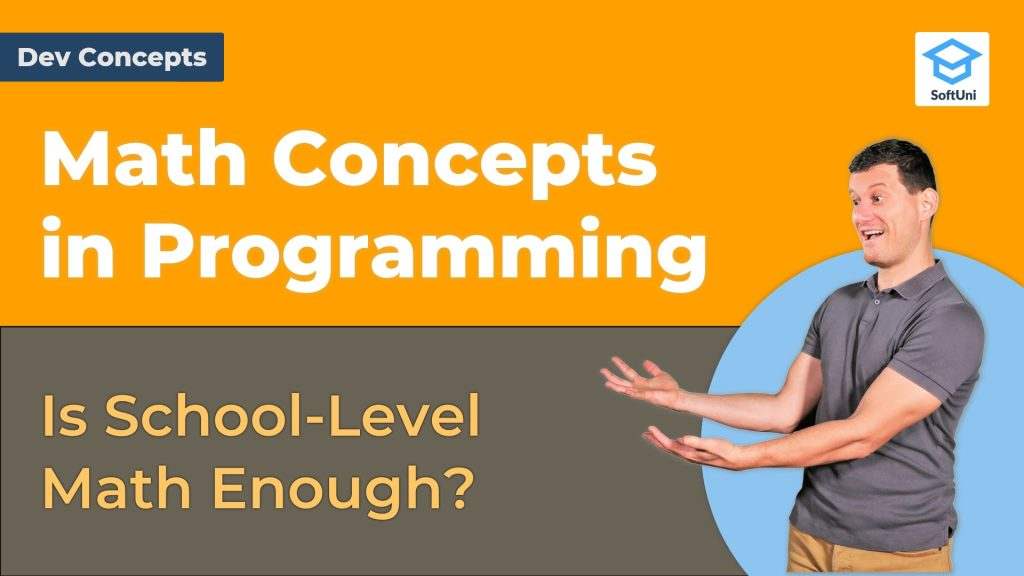# Math Concepts in Programming [Dev Concepts #2]

Do you need math to be a programmer? Is school-level math enough? Find out in this video!Have you ever wondered whether you need to be good at math to become a developer? Well, in this video we explain it all. Watch it to find out just how much math developers use daily!

Simple mathematical concepts are often needed and applied in software development.

This doesn’t mean that software developers should be strong mathematicians, but they should be familiar with the basic math concepts at school level.

Developers don’t need to prove theorems or solve differential equations, but they need to understand coordinate systems, they need to know what a function is, they should be able to perform simple calculations, and they should have well-developed algorithmic thinking, which is like the logical thinking used in math.

School-level math is enough for most developers, unless they are involved with computer graphics, machine learning or other math-intensive specialization.

## Lesson Topics

Below are the most important mathematical concepts related to programming:

• Coordinate systems and graphical objects with their size, position, and other numerical characteristics are used in computer graphics and user interface systems, such as HTML and CSS, PDF, SVG and others.
• Mathematical functions, elements of lambda calculus, discrete functions and other simple mathematical transformations are used by developers when they write code, as part of the functional programming paradigm and some modern architectural approaches like “serverless computations”. For example, developers may define functions in their programming language, which transform input values into an output result. Or they can pass a function (called a callback or delegate) as a parameter to other function.
• Vectors and matrices may be used in graphics, machine learning, and everywhere else. In fact, developers use vectors every day, because “vector” is the mathematical name of “arrays and lists”. “Matrices” is the mathematical name of “tables” that we know well from spreadsheet and Excel applications.
• Finite state automata and state machines are used in parsers (which extract data from structured text), in-process management and workflow management software, and in interpreters and compilers.
• Statistics concepts, such as probability distributions, statistical significance, hypothesis testing, and regression are widely used in data science and machine learning. In machine learning, data science, and artificial intelligence systems, math skills are more important. But in traditional (mainstream) Web, mobile, and back-end development, we need nothing more than school-level math and logical thinking.
• Algorithm complexity is a math concept from the mathematical analysis, used to estimate the execution speed of the code, based on the approximation of the steps needed to be executed. Developers need to learn the concept of “time complexity” and what is linear time, quadratic time, cubic time, and logarithmic time, which is quite simple, and mathematics is not deeply involved.
• Mathematical modeling is the art of translating problems from an application area into tractable mathematical or formal formulations whose theoretical, numerical, and algorithmic analysis provides insight, answers, and guidance useful for the originating application. Mathematical modeling is an important skill, related to algorithmic thinking, and developers with strong problem-solving skills learn how to use it at a basic level.